## Tuesday, November 29, 2016

### Detection of Changes in Images with 1D Haar Wavelet Spikes

Introduction

Haar Wavelet Transforms (HWTs) are used to detect significant changes in signal values. In two previous posts (here and here), I formalizied 1D Haar Wavelet up-down and down-up spikes that can be used to detect changes in signals. Specifically, four types of spikes were proposed: up-down triangle, up-down trapezoid, down-up triangle, and down-up trapezoid. In this post, I will formally outline a method for using 1D Haar Wavelet Spikes to count changes in images.

Spike Pixels

Let us assume that we will be detecting changes in image rows. Image columns will work just as well. A spike pixel then is a pixel covered by an up-down or down-up spike detected in some row. Formally, when spikes are computed for row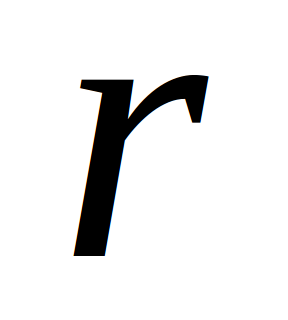, the column indices of the actual pixels covered by each spike at scale j are computed by the formula in Eq. 1, where s and e are the positions of the starting and ending wavelet coefficients in the 1D HWT at scale j, respectively. For up-down spikes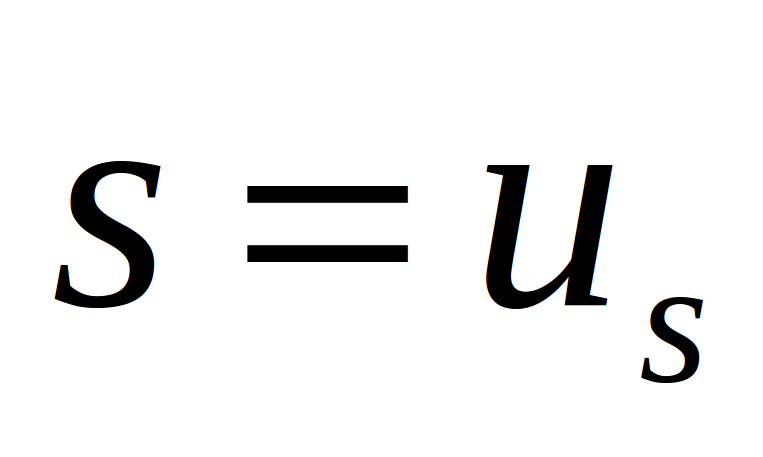and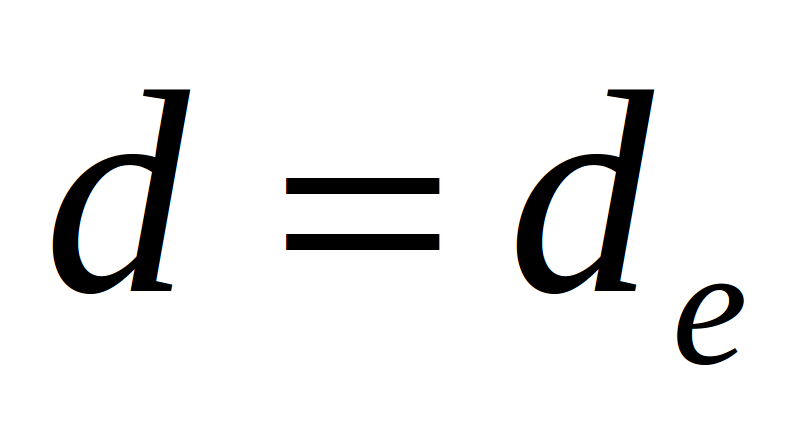, whereas, for down-up spikes,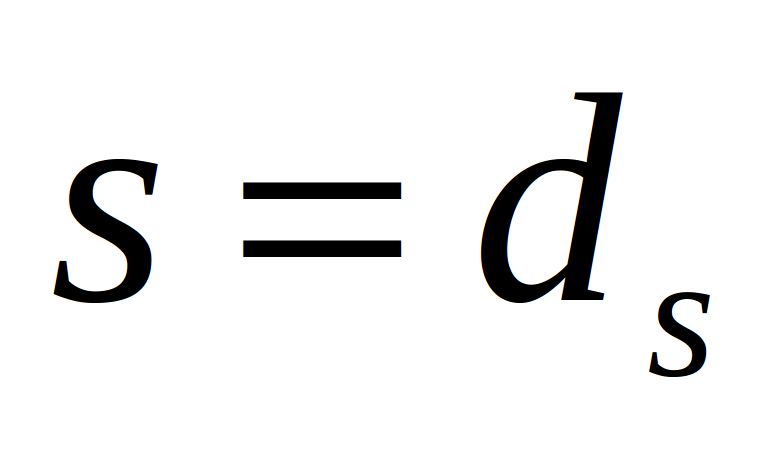and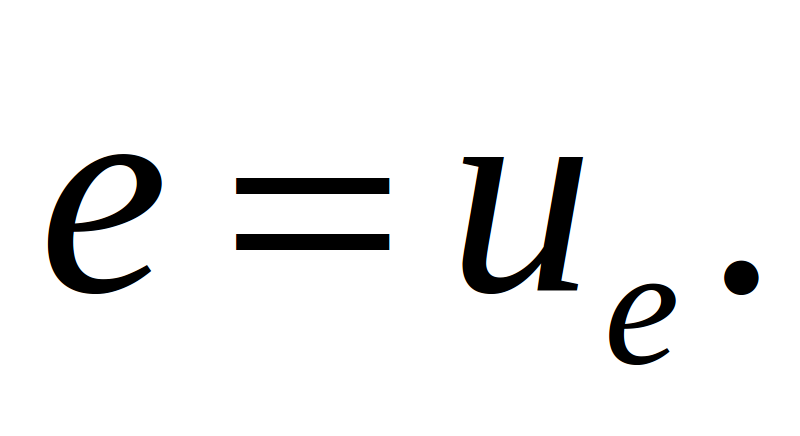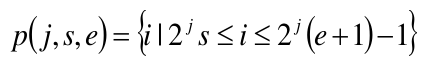Eq. 1. Column indices of pixels covered by spikes.

Let n be the number of rows in an image and let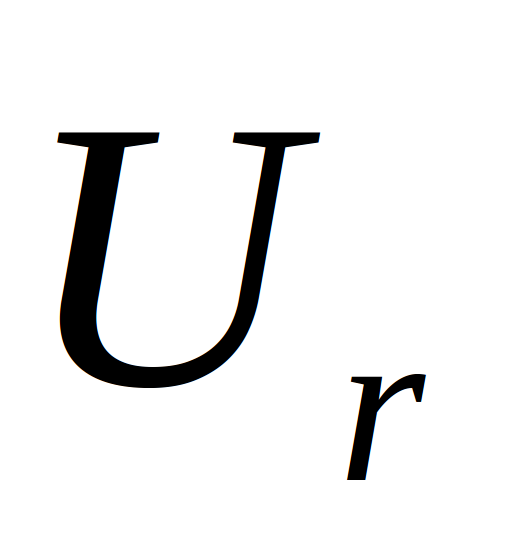be the set of up-down spikes in row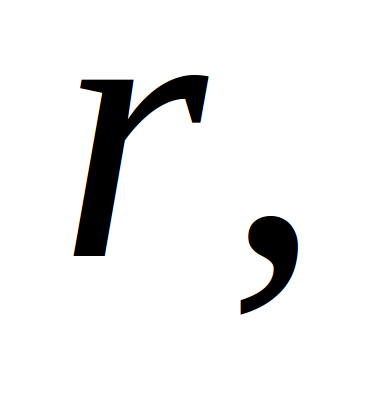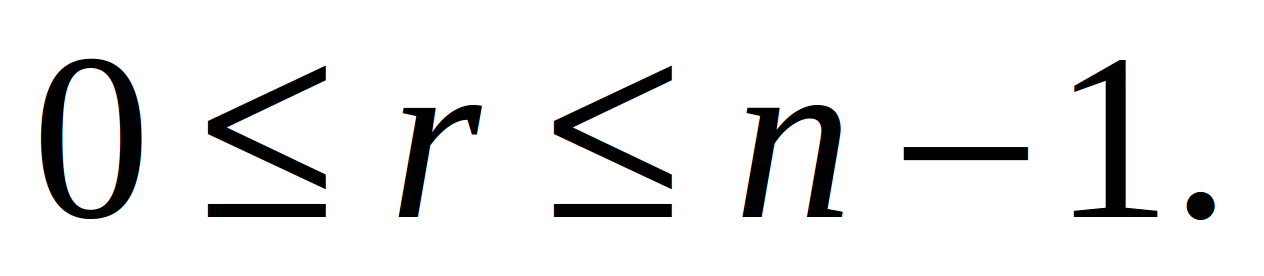Then the set of pixel columns in rowcovered by the up-down spikes inis given in Eq. 2, where j is a given scale and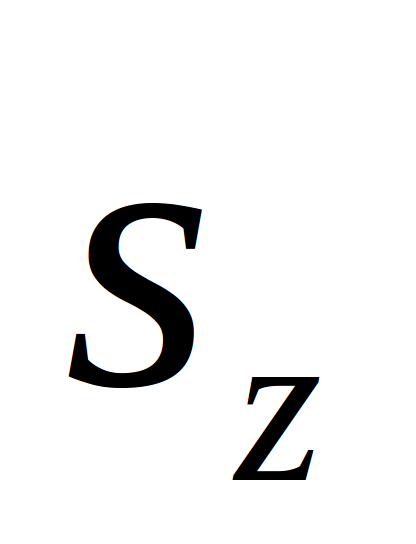and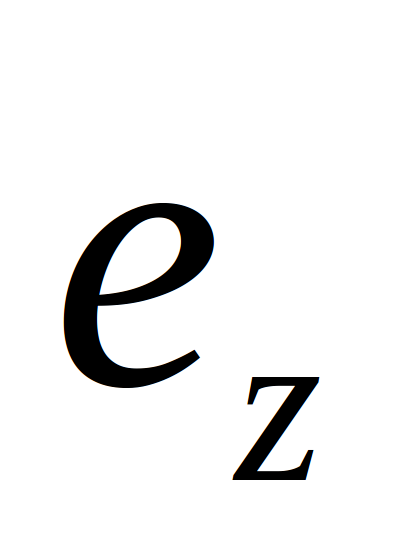are the beginning and end positions of an up-down spikedetected in rowrespectively.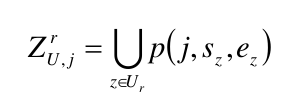Eq. 2. Set of pixel columns in rowcovered by up-down spikes.

Let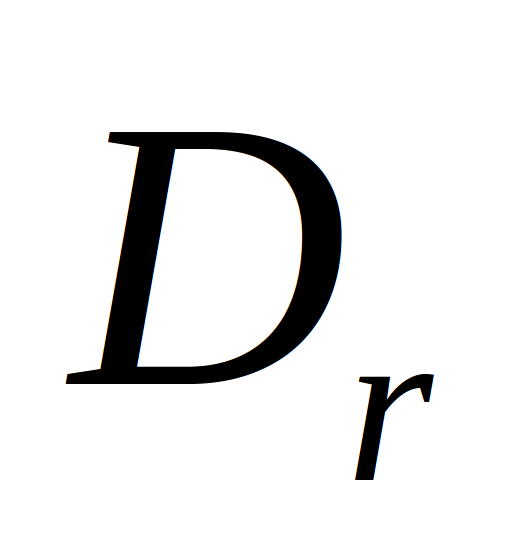is the set of down-up spikes in rowThen the set of pixel columns incovered by the down-up spikes is Eq. 3, where j is a given scale andandare the beginning and end positions of a down-up spikedetected in rowrespectively.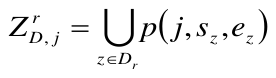Eq. 3. Set of pixel columns in rowcovered by down-up spikes.

The number of unique column pixels covered by the up-down and down-up spikes in rowis given in Eq. 4. The formula in Eq. 5 gives the actual number of pixels covered by the up-down and down-up spikes in an image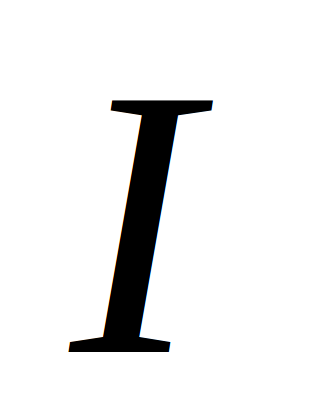with n rows.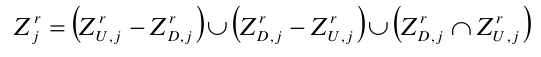Eq. 4. Set of unique pixel columns in rowcovered by down-up spikes.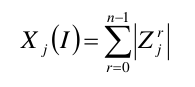Eq. 5. Number of unique pixel columns covered by up-down and down-up spikes in  imagewith n rows.